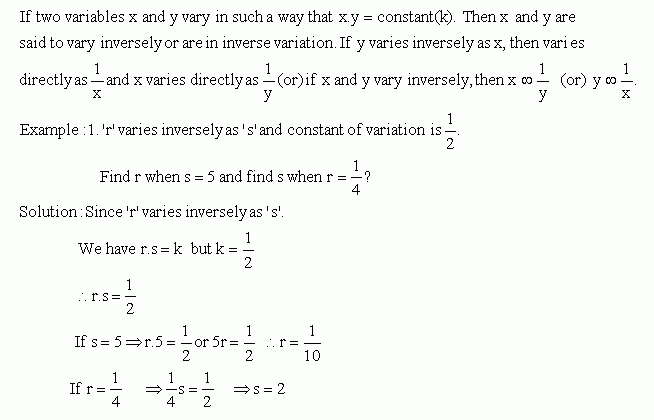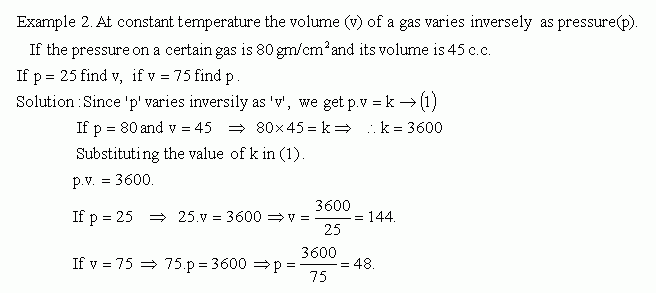Email us to get an instant 20% discount on highly effective K-12 Math & English kwizNET Programs!

#### Online Quiz (WorksheetABCD)

Questions Per Quiz = 2 4 6 8 10

### MEAP Preparation - Grade 7 Mathematics2.17 Inverse Variation or Inverse Proportion

 Inverse Variation or Indirect VariationDirections: Answer the following questions. Also write at least ten examples of your own.
 Q 1: A farmer has enough grain to feed 50 cattle for 10 days. He sells 10 cattle. For how many days will the grain last now?50 days40 days55 days Q 2: In an army camp, there is food for 8 weeks for 1200 people. After 3 weeks, 300 more soldiers joined the camp. For how many more weeks will the food last?another 4 weeksanother 3 weeksanother 5 weeks Q 3: Some people working at the rate of 6 hours a day can complete the work in 19 1/2 days. As they have received another contract, they want to finish this work early. Now they start working 6 1/2 hours a day. In how many days will they finish this work?16 days20 days18 days Q 4: If y varies inversely as x; and x=6 when y=-3, find y when x=-9.623 Q 5: It is known that current (A) in an electric circuit is inversely proportional to the resistance (R) in the circuit. When the resistance is 3 ohms, the current is 2 amperes. Find the resistance if the current is 5 amperes; and find the current when the r1 ohms and 1 amperes respectively1.2 ohms and 1.2 amperes respectively2 ohms and 1 amperes respectively Q 6: The number 'r' varies inversely as 's' and the constant of variation is 20. Find s when r=5; and find r when s=-2.4 and -10 respectively-10 and 5 respectively5 and -10 respectively-10 and 4 respectively Q 7: 24 people can construct a house in 15 days. But the owner would like to finish the work in 12 days. How many more workers should he employ?768 Q 8: The groceries in a home of 4 members are enough for 30 days. If a guest comes and stays with them, how many days will the groceries last?28 days26 days24 days Question 9: This question is available to subscribers only! Question 10: This question is available to subscribers only!